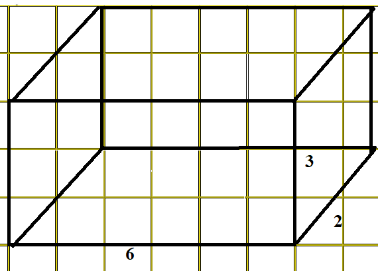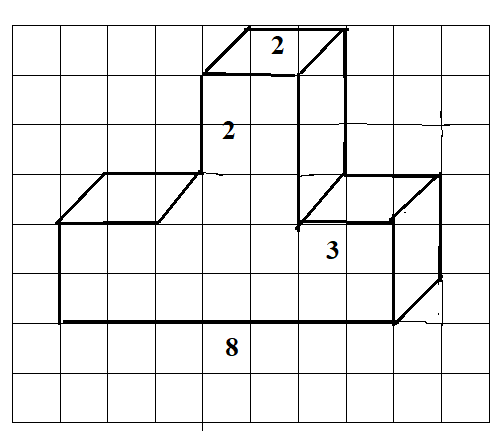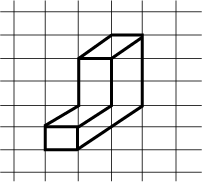Filters
Latest Questions
Mathematics
Isometric sketches
Use isometric dot paper and make an isometric sketch for each one of the given shapes:Mathematics
Isometric sketches
Draw a tetrahedron in isometric dot paper.
Mathematics
Isometric sketches
Draw (i) an oblique sketch and (ii) an isometric sketch for a cuboid of dimensions 5cm, 3cm and 2cm. (Is your sketch unique)?
Mathematics
Isometric sketches
Use isometric dot paper and make an isometric sketch for the given shape.Mathematics
Isometric sketches
The dimensions of a cuboid are 5 cm, 3 cm and 2 cm. Draw three different isometric sketches of this cuboid.
Mathematics
Isometric sketches
Make an oblique sketch for the given isometric shape below:Mathematics
Isometric sketches
Use isometric dot paper and make an isometric sketch for the given shape:Mathematics
Isometric sketches
Which term of the A.P. 3,15,27,39,....will be 120 more than its ${21^{st}}$ term?
Mathematics
Isometric sketches
${\text{Which term of the }}A.P.\;3,15,27,39,....{\text{will be }}120{\text{ }}{\text{more than its }}{21^{st}}{\text{term}}?$
Prev
1
Next